(12000 SR)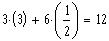Therefore, cost of three computers and six printers will be:
cost of one printer = 1/2 (500 SR)
cost of one computer = 3 (3000 SR)
==>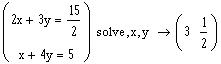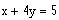and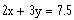==>
Let x = cost of computers and y = cost of printers
Solution:
e)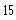d)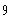c)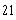b)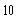a)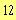7. (001-Final-7 \$9.1) I The cost in thousands of SR of two computers and three printers is 7.5 and the cost of one computer and four printers is 5, then the cost of three computers and six printers is:
Section 9.1
A. M. Farhat
Solution of Old Exams Problems

Chapter 9
Math002